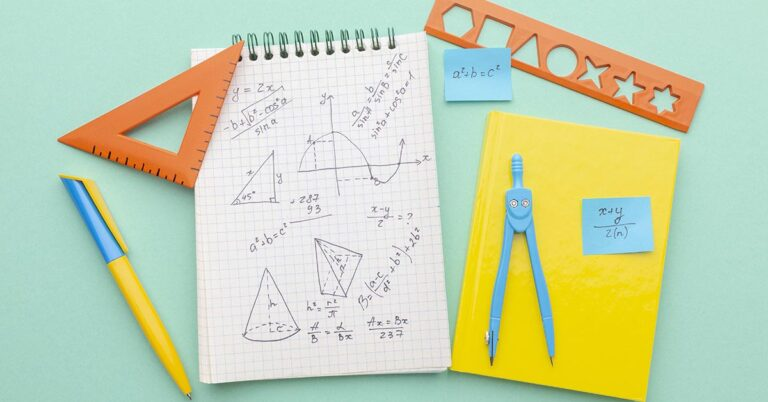# PSLE math 2023 questions answeredNow that the PSLE Math 2023 exam is over, you might be wondering how difficult this year’s math questions are. Let’s take a peek at a few of these PSLE questions from Paper 1 and 2 and learn how to answer them.

The 2 PSLE math questions that we are going to look at are as follows:

## 2023 PSLE Math question on speed | Paper 1

In this P6 speed question, we are given that Faizal and Elise started jogging from the same spot at the same time. Faizal’s speed was 30 m/min faster than that of Elise’s. Both of them did not change their speed throughout the jog. Faizal jogged all the way to the end of the track and immediately made a U-turn. They passed each other at the 4000-m mark of the track. We then need to solve for Elise’s speed.

### How to solve this paper 1 math question

Here’s the answer to this math problem:You can solve this math problem easily with the help of some patience and the speed-distance-time formula. The trick is to see the relationship between the extra distance that Faizal jogged and his speed relative to Elise’s.

## PSLE Math 2023 question in paper 2 | Area and perimeter

In this math problem, we are given a trapezium with a perimeter of 96 cm and there are 2 parts to the question.

In part a, we need to look at a figure that is made up of 3 such trapeziums with a perimeter of 204 cm. We are then asked to find the length AB.

In part b, we are given another figure that is  made up of 4 such trapeziums which form 2 identical rectangles. Given that the total area of these rectangles is 1932 cm2m we’ll need to solve for the length AD.

Here’s the solution to this problem!

### How to solve this paper 2 questionThis question may seem difficult at first, but it can be solved by applying what you’ve learnt about area and perimeter in Primary 3.